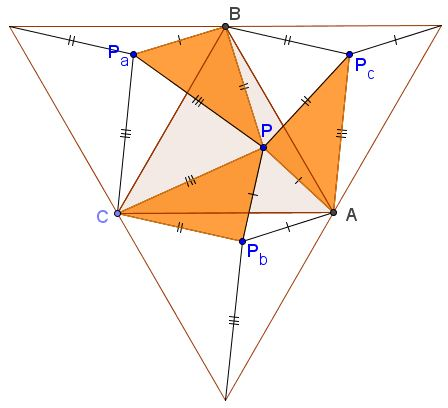# Equilateral and 3-4-5 Triangles

In an equilateral triangle ABC a point P is found such that AP = 3, BP = 4, and CP = 5. Find the side of ΔABC.

29 April 2015, Created with GeoGebra

SolutionIn an equilateral triangle ABC a point P is found such that AP = 3, BP = 4, and CP = 5. Find the side of ΔABC.

The problem resembles one in a square, where a point was joined to three vertices of the square with segments of lengths 3, 4, and 5. In addition, there had to be significance to the lengths being the sides of the 3-4-5 triangle. The applet below illustrates the solution.Rotate ΔABC 60° around each of its vertices, all in the same direction. This will produce and equilateral triangle consisting of 4 copies of ΔABC, with three images of point P: Pa, Pb, Pc. The area of hexagon APcBPaCPb can be evaluated in two ways.

First, note that the complement of the hexagon in the big triangle consists of copies of triangles ABP, BCP, and CAP, each appearing twice. It follows that Area(APcBPaCPb) = 2 Area( ΔABC).

Also, the hexagon is the union of three equilateral triangles, with sides 3, 4, 5, and of three 3-4-5 triangles, which we know to be right by the converse of the Pythagorean theorem. We see that

2 Area(a) = Area(3) + Area(4) + Area(5) + 3×(3·4/2),

where Area(x) denotes the area of an equilateral triangle with side x and a is the unknown side of ΔABC. Since

A(x) = x²3/4,

we are led to the following equation:

2 a²3/4 = (3² + 4² + 5²)3/4 + 18,

solving which we find that

a² = (25 3/4 + 9) 4 / 3.• Rusty Compass Construction of Equilateral Triangle
• Equilateral Triangle on Parallel Lines
• Equilateral Triangle on Parallel Lines II
• When a Triangle is Equilateral?
• Viviani's Theorem
• Viviani's Theorem (PWW)
• Tony Foster's Proof of Viviani's Theorem
• Viviani in Isosceles Triangle
• Viviani by Vectors
• Slanted Viviani
• Slanted Viviani, PWW
• Morley's Miracle
• Triangle Classification
• Napoleon's Theorem
• Sum of Squares in Equilateral Triangle
• A Property of Equiangular Polygons
• Fixed Point in Isosceles and Equilateral Triangles
• Parallels through the Vertices of Equilateral Triangle
•